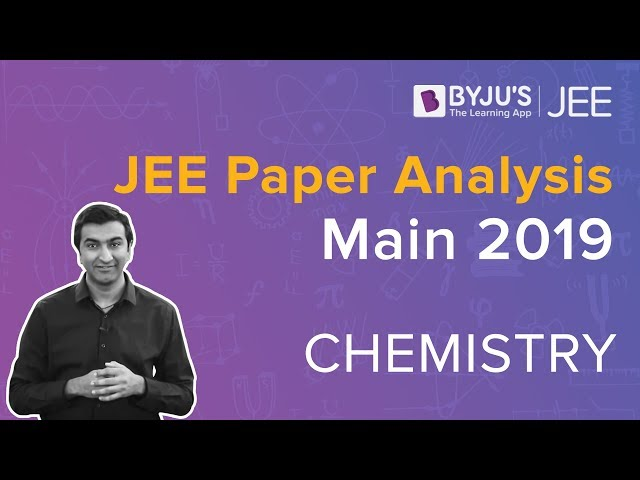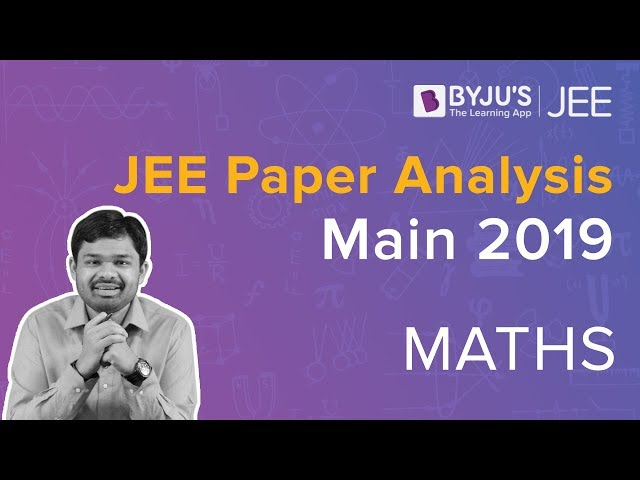# JEE Main 2019 Paper Analysis

The two sessions of JEE Main 2019 were held in the months of January and April. Approximately, 11 lakhs of candidates appeared for the entrance exam and each of them had different views about the question paper. As far as the students’ reaction go some said that Maths was the most difficult section while others were of the opinion that Physics was tougher and lengthy. Chemistry, as usual, was the easiest section for everyone.

However, to present only the precise data our experts have carefully reviewed the papers and they have provided a comprehensive analysis of the 2019 JEE Main papers. They have explained how the questions were set, from which chapters the questions were asked and the overall difficulty level. Engineering aspirants can take in all the information that is provided and significantly boost their JEE preparation.

## JEE Main 2019 Overview

JEE Main 2019 question paper pattern is given here. A total of 90 questions (4 marks each) were asked based on the syllabus prescribed by NTA for Physics, Chemistry and Maths subjects.

 Specifications JEE Main Question Paper 2019 Exam Mode Computer-Based Online Test Exam Duration 3 hours Subjects PCM Total Marks 360 Marks Question Type Multiple choice single correct answer For correct Answers +4 marks For incorrect Answers -1 mark

Candidates can also get a hold of the JEE Main 2019 question paper by visiting the given link.

Download Analysis As PDF: JEE Main 2019 Paper Analysis PDF

## JEE Main 2019 Paper 1 Analysis

#### JEE Main 2019 Chemistry Analysis#### JEE Main 2019 Maths Analysis### JEE Main 2019 Question Paper 1

JEE Main 2019 Question paper 1 is basically set for students looking to pursue B.E. and B.Tech courses. After reviewing the question, our experts have suggested that the overall difficulty of the paper was moderate and had evenly balanced marking scheme for the subjects such as maths, physics and chemistry. However, as per the feedbacks physics was the most difficult section while maths was moderate and chemistry was fairly easy to solve.

 Subject Difficulty Scale Physics Was difficult with tricky questions. Maths Was moderate to difficult with the majority of the questions based on the topic of integration. Chemistry Comparatively easy and scoring.

Well, will look at the subject wise question below.

### JEE Main 2019 Question Paper Physics Section

Physics, as usual, was the toughest section in JEE Main 2019 paper with tricky questions that further required more logical and reasoning skills. The section was allotted a total of 30 marks where 13 questions were set from electromagnetism, 8 from mechanics 2 from modern physics, 3 from optics, 3 from thermodynamics,1 from SHM and Waves. In this paper, 10 questions were quite easy, 17 were moderate and 3 questions were difficult.

### JEE Main 2019 Question Paper Maths Section

The questions that were asked in the mathematics section was surprisingly on a moderate level. Candidates who appeared for the exam stated that the questions involved a few calculations which took a bit longer time to solve and attempt all the questions. There were 9 questions from algebra, 8 from coordinate geometry, 9 from calculus and 4 were based on probability, mathematical reasoning and statistics. Overall, 13 questions were fairly easy, 13 were on a moderate level and 4 questions were difficult.

### JEE Main 2019 Question Paper Chemistry Section

Chemistry section was the easiest and scoring section among all the three sections. There was equal weightage given to inorganic, physical and organic chemistry. However, class 12 was given more importance as 17 questions were asked while 13 questions were asked from class 11 syllabus. There were 12 questions from physical chemistry, 10 from organic chemistry and 8 from inorganic chemistry. Out of these, 11 questions quite easy, 16 on a moderate level and 3 required a bit of thinking but was answerable. Students who have performed well in this section will surely have a greater chance to excel and advance forward.

### JEE Main 2019 Paper 2 Analysis

In paper 2 the exam was conducted both in a computer-based mode as well as pen and paper-based mode. Mathematics and General Aptitude test was conducted online whereas the drawing test was conducted in offline mode.

Maths Section:

The question in the mathematics section was difficult. Majority of the questions were based on the topic of calculus. Interestingly, the questions that appeared in the April paper did not bear any similarity to the questions that were asked in the January session.

General Aptitude Section:

General Aptitude section consisted of tricky questions. There were questions based on topics such as identifying 3D objects and shapes. There were general knowledge questions as well and candidates were asked details about the location of places which had architectural importance. They were also asked the full form of important words and more. There was a total of fifty questions which carried four marks each.

Drawing Section:

As for the drawing section it consisted of easy questions. However, students were of the opinion that the questions were lengthy and required more time to solve them. There were 3 questions which carried a total of seventy marks. The questions were basically designed to test the creativity and drawing skills of the students.

### JEE Main 2019 Paper Physics, Maths and Chemistry Chapterwise Questions

Below students will find the number of questions that were asked from the different chapters in the three sections.

 Maths Topics No of Questions Weightage of Marks Sets, Relation and Function 1 4 Sequences and Series 1 4 Binomial Theorem and Its Application 1 4 Differential Equation 1 4 Differential Calculus 1 4 Permutations and Combinations 1 4 Trigonometry 1 4 Mathematical Reasoning 1 4 Statics and Dynamics 1 4 Statistics and Probability 2 8 Matrices and Determinants 2 8 Vector Algebra 2 8 Three Dimensional Geometry 2 8 Complex numbers and Quadratic Equation 2 8 Limits, Continuity and Differentiability 3 12 Integral Calculus 3 12 Coordinate Geometry 5 20 Total 30 120

 Physics Topics No of Questions Weightage of Marks Electromagnetics Induction; AC 1 4 Solids and Fluids 1 4 Waves 1 4 Work, Power and Energy 1 4 Gravitation 1 4 Simple Harmonic Motion 1 4 Unit, Dimension and Vector 1 4 Kinematics 1 4 Laws of motion 1 4 Centre Of Mass, Impulse and Momentum 1 4 Rotation 1 4 Magnetics 2 8 Heat and Thermodynamics 3 12 Current Electricity 3 12 Electrostatics 3 12 Optics 3 12 Modern Physics 5 20 Total 30 120

 Chemistry Topics No of Questions Weightage of Marks Electrochemistry 1 4 General Organic Chemistry 1 4 Stereochemistry 1 4 Carbohydrates, amino acid and Polymers 1 4 Alkyl Halides 1 4 Carboxylic Acid and their Derivatives 1 4 Aromatic Compounds 1 4 Redox Reaction 1 4 Mole Concept 1 4 Solution and Colligative Properties 1 4 Hydrocarbon 1 4 Chemical Kinetics 1 4 Solid-State And Surface Chemistry 2 8 Thermodynamics And Gaseous State 2 8 Chemical Bonding 2 8 Chemical And Ionic Equilibrium 2 8 Atomic Structure 2 8 Nuclear Chemistry And Environment 2 8 The periodic table and Representative Elements 3 12 Transition Elements and Coordination Chemistry 3 12 Total 30 120

## JEE Main Year-wise Paper Analysis

Candidates can also check the JEE Main analysis for 2018, 2017, 2016,2015, 2014, 2013 below.